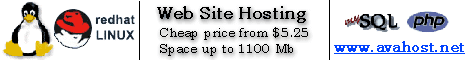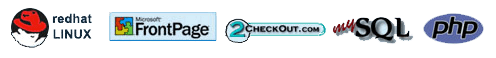Cheap Web Hosting
Reviews and Articles
low cost high quality hosting
 Cheap Web Hosting Reviews and Articles
 Cheap Web Hosting Web Hosting Reviews Articles FAQ Recommended Web Hosting
 OptionsCheap Web HostingWeb Hosting ReviewsArticlesFrequently Asked QuestionsRecommended Web HostingLinksWeb Hosting Articles
Conceptual bubble sort theory

As a programmer, it is inevitable that at some point you will be faced with the task of sorting data regardless of the language you are using. A school system may want their student data sorted, same with a business's payroll book or a bank's transaction logs. The bubble sort is the simplest method of sorting array data known to man at this point. By the end of this article, you will understand the concept of the bubble sort and even understand code demonstrating the task.

Bubble sorting is conceptually easy to understand. It looks at two positions within an array, compares the two, and makes any changes it needs. Let's look at a set of random numbers.

For those unfamiliar with Arrays

Before we continue, if you are not familiar with arrays, you will be lost, so allow me to take a moment and explain the concept of arrays. Simply put, arrays are datatypes that can hold more than one value. So, instead of Num holding one value, if it is an array, it could hold many. Let's look at a quick example.

int Num={10, 12, 14, 16, 18, 20};

This array would look something like this:

 0 1 2 3 4 5 10 12 14 16 18 20

Since the number 10 was defined first, it will rest in cell position 0, 12 was defined next, so it will rest in cell position 1, and so on.

We work with values within arrays by the cell position of the value. So, if we wanted to work with the 10, we'd call Num. If we wanted to work with 20, we'd call Num. Get it?

 5 34 32 25 75 42 22 2 --- 5 32 34 25 75 42 22 2 --- 5 32 25 34 42 22 2 75 We are tasked with sorting these 8 numbers to the left in ascending fashion, that is, from the smallest to the largest. Obviously, they are not yet in that order, so we need to write a little bit of code to accomplish this task. First though, let's look at this using English. To begin, we look at the first two numbers, 5 and 34. Since we are sorting in an ascending fashion, the smallest number should be first. Is it? Yes, so for the first two numbers, no changes need to be made. Next, we look at the second and third numbers, 34 and 32. Oops, a change needs to be done here, right? Since 32 is under 34, we need to switch the two numbers. So, we'll switch them. So now the numbers look like the second set on the left. Continuing, we look at 34 and 25. Yes, we need to switch them. Then we look at 34 and 75. No, no changes are needed. 75 and 42 need changing, 75 and 22 need changing and 75 and 2 need changing. So, our set of numbers now looks like the third set on the left. So, we've gone through the list once and they still aren't sorted. What do we do now? Well, we loop through the list again and perform the same procedure. Notice the original list of numbers had the smallest number, 2, at the bottom so we need to loop through the number of items in the array - 1. Why? When we loop through the array, we instruct the program to look at the current array position and also the current array position + 1. It is better explained when accompanied by code, so let's take a look at what code would look like to pull this sort off.

There are a number of languages we can demonstrate this in, but I have selected the one most used by computer programmers these days, C++. If you have a C++ compiler, you can even copy and paste this code and see it run for you. Let's take a look at the entire code first, then I'll explain line by line what the code means.

``` #include <iostream> using std::cout; using std::endl; #include <iomanip> using std::setw; void main() {     int NumList = {5, 34, 32, 25, 75, 42, 22, 2};     int Swap; cout<<"Data in original order: \n"; // This line loops through the array and displays all values, as is for(int ctr=0; ctr<8; ctr++) {     cout<< setw( 3 ) <<NumList[ctr]; }     cout<<"\n\n"; // Loop through the array one less than its total cell count for(int i=0; i<7; i++) // Loop through every cell (value) in array      for(int ii=0; ii<7; ii++) // Compare the values and switch if necessary        if (NumList[ii] > NumList[ii + 1])        {        Swap = NumList[ii];        NumList[ii] = NumList[ii + 1];        NumList[ii + 1] = Swap;        } cout<<"Data in ascending order: \n"; // Print out sorted array for (int iii=0; iii<8; iii++) cout<< setw( 3 ) << NumList[iii]; cout<< endl <<endl; } ```

Okay, let's go through this code.

``` #include <iostream> using std::cout; using std::endl; ```

We first must include a library file so we can output the appropriate arrays to the video.

``` #include <iomanip> using std::setw; ```

We must also include this file so we can set the spaces on the array data. Notice we used setw( 3 ) when we displayed the array. The 3 refers to the spaces we step before we display the value.

``` void main() {     int NumList = {5, 34, 32, 25, 75, 42, 22, 2};     int Swap; ``` Here we simply define our function, called main, and initialize an array NumList with 8 array cells. Within the curly braces we have defined our 8 values. On the next line we define our Swap variable, which will temporarily hold our value while we switch array positions.

``` cout<<"Data in original order: \n"; for(int ctr=0; ctr<8; ctr++) {     cout<< setw( 3 ) <<NumList[ctr]; }     cout<<"\n\n"; ``` Here we simply write a for loop to iterate through each array position and display its value. We have set a space count at 3 and we put in two line breaks after our print out.

``` for(int i=0; i<7; i++) ``` This for loop will control how many times we look through the entire list of array values. Although our array has 8 values, we must keep the array at < 7 because of our progression through the array. More on that later.

```      for(int ii=0; ii<7; ii++)        if (NumList[ii] > NumList[ii + 1])        {        Swap = NumList[ii];        NumList[ii] = NumList[ii + 1];        NumList[ii + 1] = Swap;        } ``` Here we have our second loop. This will iterate through each position of the cell and the if statement will compare both values. if NumList[ii] > NumList[[ii + 1] means that if the current position is greater than the next position, Swap = NumList[ii], we will assign the value in NumList[ii] to Swap so we will not lose that data when we do the switch. Then, we assign NumList[ii] to the value of NumList[ii + 1]. Lastly, we assign Swap, which was the current position, NumList[ii], to the next position, or NumList[ii + 1].

``` cout<<"Data in ascending order: \n"; for (int iii=0; iii<8; iii++) cout<< setw( 3 ) << NumList[iii]; cout<< endl <<endl; ``` Lastly, after the sort, we will display the array. Note, if you are running this C++ program on your own system, you will see all the numbers are appropriately sorted. Also note that if two numbers are the same values, like 4 and 4, no swapping will occur.

Naturally, programmers desire the fastest execution of their programs, especially while sorting. Bubble sorting is often the quickest method to choose, but with many thousands of records, sorting can become slow and inefficient, and alternative methods of sorting should be considered.

Articles from WebSiteGravy.comCopyright (c) CheapWebHosting 2002